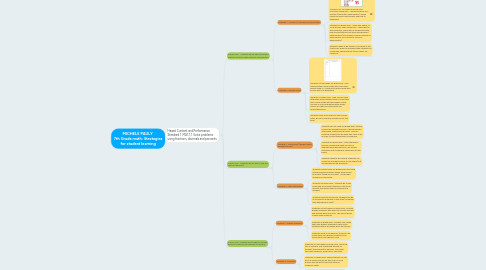# MICHELE PAULY 7th Grade math: Strategies for student learningMichele Pauly
Get Started. It's FreeMICHELE PAULY 7th Grade math: Strategies for student learning## 1. Hawaii Content and Performance Standard 1 MA7.1.1 Solve problems using fractions, decimals and percents.

### 1.1. OBJECTIVE: Students will be able to add and subtract fractions with different denominators

1.1.1. Strategy 1: Connect to background knowledge.

1.1.1.1. Students not yet ready for grade level: pre-teach vocabulary - identifying parts of a fraction (numerator, denominator), define lowest common denominator and how to determine.

1.1.1.2. Students on grade level: "Think-pair-share", in small groups, share vocabulary - which part is the numerator, which part is the denominator, how to add/subtract fractions with different denominators (find lowest common multiple of denominator and convert to common denominator).

1.1.1.3. Students ready to go beyond: Can assist in the "think-pair-share" by quizzing other students on vocabulary, explaining any terms others are unsure of.

1.1.2. Strategy 2: Guided notes

1.1.2.1. Students not yet ready for grade level: Give preformatted Cornell notes with main ideas already filled in. Students to follow along with lecture and fill in definitions.

1.1.2.2. Students on grade level: Take Cornell notes with either preformatted notes or make their own Cornell notes with the proper format. Column on left has questions/key words, column on right has notes about key points/definitions.

1.1.2.3. Students ready to go beyond: Take Cornell notes, go on to practice problems from text book.

### 1.2. OBJECTIVE: Students will be able to add and subtract decimals.

1.2.1. Strategy 1: Think aloud (teacher model thought process)

1.2.1.1. Students not yet ready for grade level: Teacher models the thought process of going through adding and subtracting decimals. This will allow the students to see what they have to do in order to solve these types of problems.

1.2.1.2. Students on grade level: After listening to teacher modeling thought process of adding/subtracting decimals, will answer questions about problems being done on the board.

1.2.1.3. Students ready to go beyond: Students can model the thought process on the board (just like the teacher did previously.

1.2.2.1. Students not yet ready for grade level: Start with simple example problems which show proper procedure (lining up decimals). Go through examples on the board.

1.2.2.2. Students on grade level: Students go to the board and do problems that are a little more difficult and explain their procedures and answers.

1.2.2.3. Students ready to go beyond: Students can go on to practice problems in text book (complete their homework in class).

### 1.3. OBJECTIVE: Students will be able to convert decimal to percent and percent to decimal.

1.3.1. Strategy 1: Graphic organizer

1.3.1.1. Students not yet ready for grade level: Provide graphic organizer with steps to convert percent and decimal back and forth. This will be glued in their math notebook.

1.3.1.2. Students on grade level: Students can create their own graphic organizer in their math notebook with information given by teacher.

1.3.1.3. Students ready to go beyond: Students can create their own graphic organizer from information from the text book.

1.3.2. Strategy 2: Chunking

1.3.2.1. Students not yet ready for grade level: Break up into 2 sections; first converting decimal to percent, then percent to decimal. Start with very easy problems, allow use of calculator.

1.3.2.2. Students on grade level: These students can still do it in chunks but will be able to do it more quickly and will get to the more difficult problems faster.

1.3.2.3. Students ready to go beyond: These students can still do it in chunks but will get to more difficult problems faster and can complete homework problems in class.